Trailing the Tail

More of a tactical, rather than practical; approach for monitoring our elephants in wild.

IntermediateFull instructions providedOver 3 days748Things used in this project

Hardware components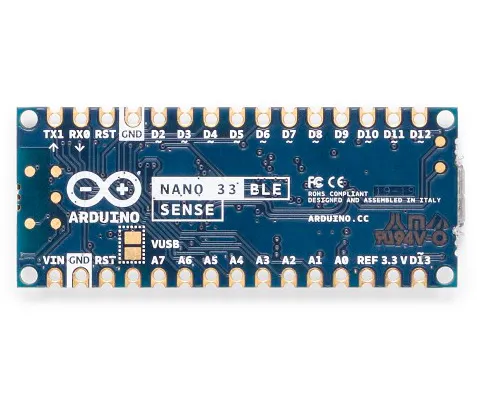Arduino Nano 33 BLE Sense
×1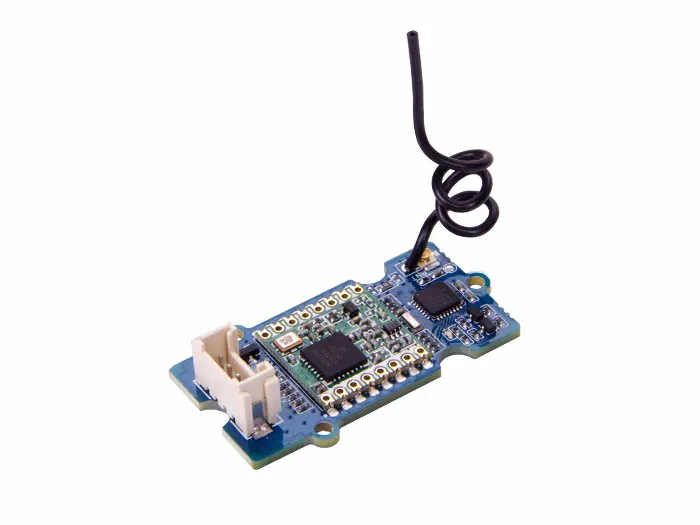Seeed Grove - LoRa Radio 868MHz
×1
 u-blox Ublox NEO-6M
×1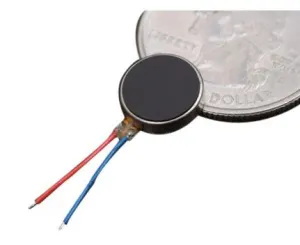Brown Dog Gadgets Solar Cockroach Vibrating Disc Motor
×1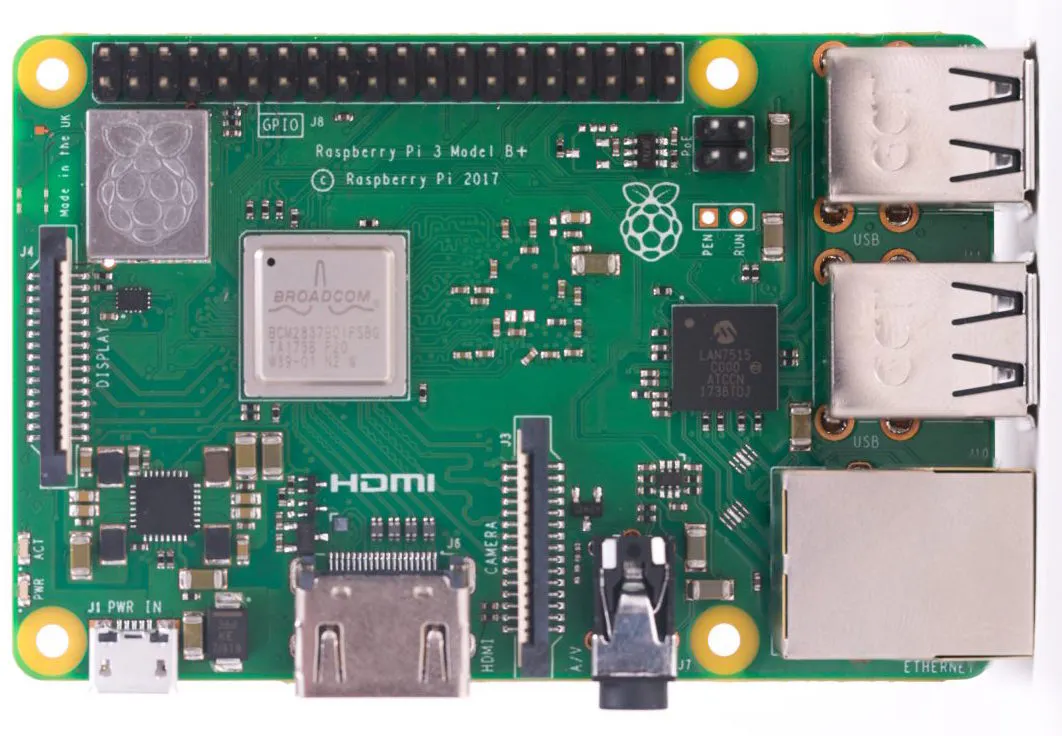Raspberry Pi 3 Model B+
×1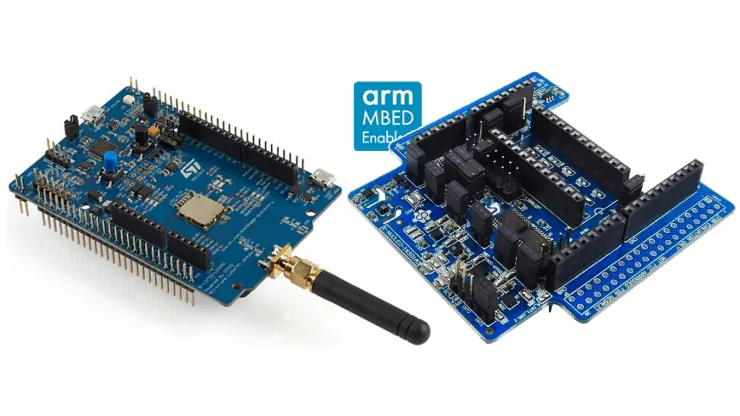Helium Developer Kit
×1
 RTL SDR dongle
×1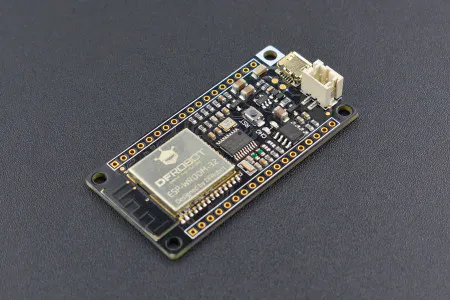DFRobot FireBeetle ESP32 IOT Microcontroller (Supports Wi-Fi & Bluetooth)
×1
 868 MHz LoRa External Antenna
×1
 GSM/CDMA External Antenna
×1
 Lithium Thionyl Chloride Battery
×3

Software apps and online servicesArduino IDE
 Avnet IoT ConnectEdge Impulse StudioIBM Watson

Hand tools and fabrication machines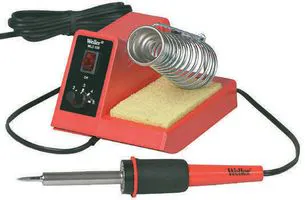Soldering Station, Hobbyist

Schematics

Connection block diagram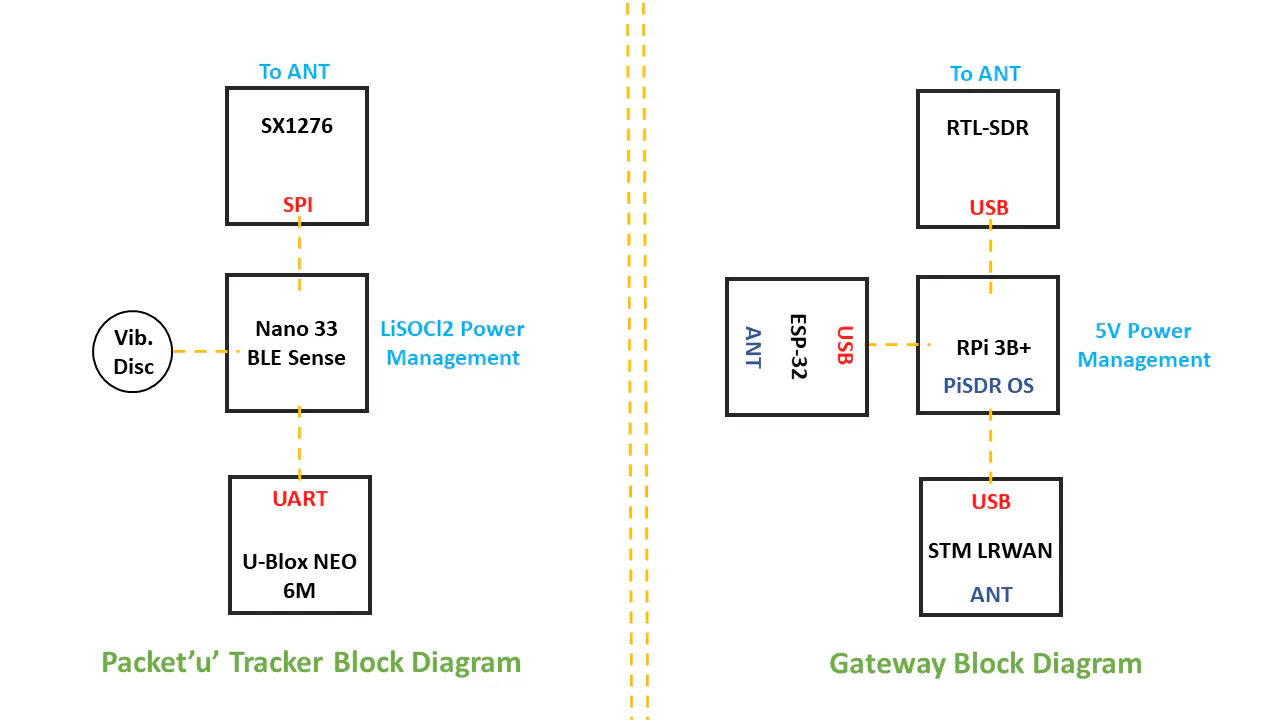Code

Packet'u' Tracker Node

Arduino
// Packet'u'[ElephantEdgeNode].
#define DECLINATION +0.36 // Target location-specific.
#include <Arduino_LSM9DS1.h>
#include <Arduino_HTS221.h>
#include <Arduino_APDS9960.h>
#define GPSSerial Serial1
#include <LoRa.h>
// Set GPSECHO to 'false' to turn off echoing the GPS data to the Serial console
// Set to 'true' to debug and listen to the raw GPS sentences
#define GPSECHO false
int EID = 31;
float temperature;
uint32_t timer = millis();
float yaw, roll, pitch;
float X, Y, Z, l, m, n, r;
int R, G, B, avg;

void setup()
{
Serial.begin(115200);
GPS.begin(9600);
LoRa.begin(865E6);
IMU.begin();
HTS.begin();
APDS.begin();
pinMode(3, OUTPUT); //HapticsEnabled.
// uncomment this line to turn on RMC (recommended minimum) and GGA (fix data) including altitude
GPS.sendCommand(PMTK_SET_NMEA_OUTPUT_RMCGGA);
// uncomment this line to turn on only the "minimum recommended" data
//GPS.sendCommand(PMTK_SET_NMEA_OUTPUT_RMCONLY);
GPS.sendCommand(PMTK_SET_NMEA_UPDATE_1HZ);
GPS.sendCommand(PGCMD_ANTENNA);
delay(1000);
GPSSerial.println(PMTK_Q_RELEASE);
}

void loop()
{
// read data from the GPS in the 'main loop'
if (GPSECHO)
if (c) Serial.print(c);
if (!GPS.parse(GPS.lastNMEA()))
return;
}
// every 2 seconds, print out the current position & stat
if (millis() - timer > 10000) {
timer = millis(); // reset the timer
Serial.print("\nTime: ");
if (GPS.hour < 10) { Serial.print('0'); }
Serial.print(GPS.hour, DEC); Serial.print(':');
if (GPS.minute < 10) { Serial.print('0'); }
Serial.print(GPS.minute, DEC); Serial.print(':');
if (GPS.seconds < 10) { Serial.print('0'); }
Serial.print(GPS.seconds, DEC); Serial.print('.');
if (GPS.milliseconds < 10) {
Serial.print("00");
} else if (GPS.milliseconds > 9 && GPS.milliseconds < 100) {
Serial.print("0");
}
Serial.print(GPS.milliseconds);
Serial.print("\tDate: ");
Serial.print(GPS.day, DEC); Serial.print('/');
Serial.print(GPS.month, DEC); Serial.print("/20");
Serial.println(GPS.year, DEC);
Serial.print("Fix: "); Serial.print((int)GPS.fix);
//Serial.print(" quality: "); Serial.println((int)GPS.fixquality);
if (GPS.fix) {
Serial.print("\tLocation: ");
Serial.print(GPS.latitude, 4); Serial.print(GPS.lat);
Serial.print(", ");
Serial.print(GPS.longitude, 4); Serial.println(GPS.lon);
Serial.print("Speed (knots): "); Serial.print(GPS.speed);
//Serial.print("Angle: "); Serial.println(GPS.angle);
//Serial.print("Altitude: "); Serial.println(GPS.altitude);
Serial.print("\tSatellites: "); Serial.println((int)GPS.satellites);
}
}
if (millis() - timer > 500) {
GetIMU();
GetAmbTemp();
GetAmbLight();
/*PacketizingLoRaTransmissions*/
LoRa.beginPacket();
LoRa.print("P'u':");
LoRa.print(EID);
LoRa.print(",[");LoRa.print(GPS.latitude, 4);LoRa.print(GPS.lat);LoRa.print("],[");
LoRa.print(GPS.longitude, 4);LoRa.print(GPS.lon);LoRa.print("],");
LoRa.print((int)GPS.fix);LoRa.print(",[");LoRa.print((int)GPS.satellites);LoRa.print("],[");

if (GPS.hour < 10) { LoRa.print('0'); }LoRa.print(GPS.hour, DEC); LoRa.print(':');
if (GPS.minute < 10) { LoRa.print('0'); }LoRa.print(GPS.minute, DEC); LoRa.print(':');
if (GPS.seconds < 10) { LoRa.print('0'); }LoRa.print(GPS.seconds, DEC);LoRa.print("],");

LoRa.print(temperature,0);LoRa.print("°C,");LoRa.print("[P:");LoRa.print(pitch, 2);
LoRa.print("],[R:");LoRa.print(r, 0);LoRa.print("],[B:");LoRa.print(yaw, 0);LoRa.print("],[X:");
LoRa.print(X);LoRa.print("],[Y:");LoRa.print(Y);LoRa.print("],[Z:");LoRa.print(Z);LoRa.print("],[Lux:");
LoRa.print(avg);LoRa.print("]");
LoRa.endPacket();
}
}

void GetAmbTemp() {
}

void GetAmbLight() {
if (APDS.colorAvailable()) {
}
avg = (R+G+B)/3;
}

void GetIMU() {
if ( (IMU.accelerationAvailable()) && (IMU.magneticFieldAvailable()) ){
roll = atan2(Y, Z);
pitch = atan2(-X, sqrt(Y * Y + Z * Z));
if (n == 0)
yaw = (m < 0) ? PI : 0;
else
yaw = atan2(m, n);
yaw -= DECLINATION * PI / 180;
if (yaw > PI) yaw += (PI);
else if (yaw > -PI) yaw += (PI);
pitch *= 180.0 / PI;
roll  *= 180.0 / PI;
yaw *= 180.0 / PI;
r = roll+90;
}
}

Packet'u' Scanner Gateway(ST-LRWAN)

Arduino

void setup( void )
{
Serial.begin(115200);
while (!Serial) { }

}

void loop( void )
{
if (packetSize) {
}
Serial.print(", SNR: ");
Serial.println(")");
}
}

SerialShark code for streaming .pcap file in Wireshark

Python
# Made by @xdavidhu (github.com/xdavidhu, https://xdavidhu.me/)

import serial
import io
import os
import subprocess
import signal
import time

try:
serialportInput = input("[?] Select a serial port (default '/dev/ttyUSB0'): ")
if serialportInput == "":
serialport = "/dev/ttyUSB0"
else:
serialport = serialportInput
except KeyboardInterrupt:
print("\n[+] Exiting...")
exit()

try:
canBreak = False
while not canBreak:
boardRateInput = input("[?] Select a baudrate (default '921600'): ")
if boardRateInput == "":
boardRate = 921600
canBreak = True
else:
try:
boardRate = int(boardRateInput)
except KeyboardInterrupt:
print("\n[+] Exiting...")
exit()
except Exception as e:
continue
canBreak = True
except KeyboardInterrupt:
print("\n[+] Exiting...")
exit()

try:
filenameInput = input("[?] Select a filename (default 'capture.pcap'): ")
if filenameInput == "":
filename = "capture.pcap"
else:
filename = filenameInput
except KeyboardInterrupt:
print("\n[+] Exiting...")
exit()

canBreak = False
while not canBreak:
try:
ser = serial.Serial(serialport, boardRate)
canBreak = True
except KeyboardInterrupt:
print("\n[+] Exiting...")
exit()
except:
print("[!] Serial connection failed... Retrying...")
time.sleep(2)
continue

print("[+] Serial connected. Name: " + ser.name)
counter = 0
f = open(filename,'wb')

check = 0
while check == 0:
if b"<<START>>" in line:
check = 1
print("[+] Stream started...")
#else: print '"'+line+'"'

print("[+] Starting up wireshark...")
cmd = "tail -f -c +0 " + filename + " | wireshark -k -i -"
p = subprocess.Popen(cmd, stdout=subprocess.PIPE,
shell=True, preexec_fn=os.setsid)

try:
while True:
f.write(ch)
f.flush()
except KeyboardInterrupt:
print("[+] Stopping...")
os.killpg(os.getpgid(p.pid), signal.SIGTERM)

f.close()
ser.close()
print("[+] Done.")

Credits

Night R

14 projects • 34 followers
R&D Engineer @ IoT Solutions Provider, Robotics Engineer @ SIS Corp., Passionate for Hardware hacking, 12+ years experience in programming..

Shivam Kumar Tiwari

1 project • 1 follower
Design Engineer (PCB and COMPONENT focus) Working as PCB and Circuit Design Engineer at Acme Digitek Solutions Pvt LTD from 5 years
Thanks to Luigi Cruz.